• 绕X轴旋转： 绕Y轴旋转： 绕Z轴旋转： ...ZX类变换：先绕着Zi-1（i-1坐标系）轴旋转和平移，然后绕Xi（i坐标系）轴旋转和平移 ...XZ类变换：先绕着Xi-1（i-1坐标系）轴旋转和平移，然后绕Zi（i坐标系）轴旋转和平移...
 绕X轴旋转：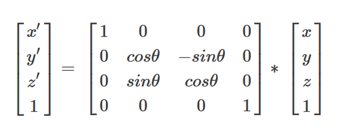绕Y轴旋转：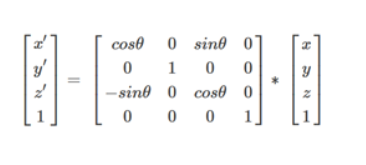绕Z轴旋转：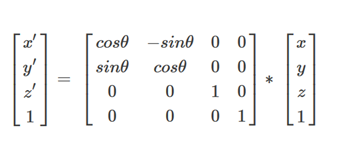SDH是ZX类变换，MDH是XZ类变换

ZX类变换：先绕着Zi-1（i-1坐标系）轴旋转和平移，然后绕Xi（i坐标系）轴旋转和平移

XZ类变换：先绕着Xi-1（i-1坐标系）轴旋转和平移，然后绕Zi（i坐标系）轴旋转和平移

SDH：变换矩阵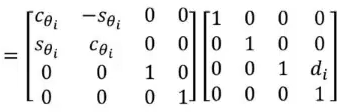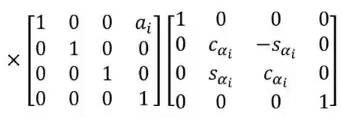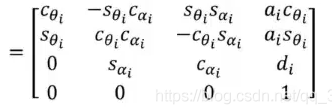MDH：变换矩阵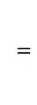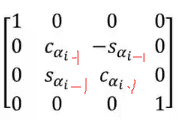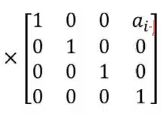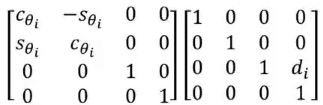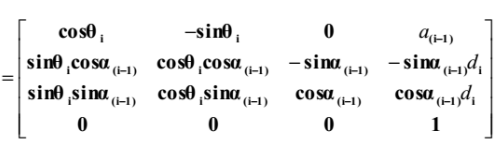展开全文• 标准DH参数描述的开放运动链的递归牛顿-欧拉逆动力学 可选的： 机器人工具箱用于比较： 下载并解压缩文件。 使用“ pathtool”命令将路径添加到MATLAB。 然后在MATLAB命令提示符下键入“ startup_rvc”。 局限性：...
• 如下参考链接1的作者大大实现了UR5机械臂的正运动学和逆运动学的...参考链接：六轴UR机械臂正逆运动学求解_MATLAB代码（标准DH参数表） UR机械臂正逆运动学求解 一、参考链接说明 参考链接2的作者实现了UR5机械.

如下参考链接1的作者大大实现了UR5机械臂的正运动学和逆运动学的Matlab代码。但逆解部分在不同版本的Matlab中运行有错误。

本篇文章是MatlabR2016a下完成的，并说明一下原代码错误的原因。

目录

一、参考链接说明

二、UR5机械臂运动学正解

三、UR5机械臂运动学逆解

四、测试代码

五、参考链接1的逆解代码错误原因

参考链接：六轴UR机械臂正逆运动学求解_MATLAB代码（标准DH参数表）

UR机械臂正逆运动学求解

一、参考链接说明

参考链接2的作者实现了UR5机械臂的正运动学和逆运动学的理论推导，给出了求解公式；

参考链接1的作者在参考链接2的基础上，写出了Matlab代码，但作者给出的代码只能在特定的Matlab中运行，如2017b。

二、UR5机械臂运动学正解

function T = zhengyundongxue(theta)
%已知关节角求变换矩阵
a=[0,-0.42500,-0.39225,0,0,0];
d=[0.089159,0,0,0.10915,0.09465,0.08230];
alpha=[pi/2,0,0,pi/2,-pi/2,0];

T01=T_para(theta(1),d(1),a(1),alpha(1));
T12=T_para(theta(2),d(2),a(2),alpha(2));
T23=T_para(theta(3),d(3),a(3),alpha(3));
T34=T_para(theta(4),d(4),a(4),alpha(4));
T45=T_para(theta(5),d(5),a(5),alpha(5));
T56=T_para(theta(6),d(6),a(6),alpha(6));

T=T01*T12*T23*T34*T45*T56;

end

function T = T_para(theta,d,a,alpha)
T=[ccc(theta),-sss(theta)*ccc(alpha),sss(theta)*sss(alpha),a*ccc(theta);
sss(theta),ccc(theta)*ccc(alpha),-ccc(theta)*sss(alpha),a*sss(theta);
0,sss(alpha),ccc(alpha),d;
0,0,0,1];
end

function sss=sss(a)
%     sss=sin(a/180*pi);
sss=sin(a);
end

function ccc=ccc(a)
%     ccc=cos(a/180*pi);
ccc=cos(a);
end

三、UR5机械臂运动学逆解

function theta=niyundongxue(T)
%变换矩阵T已知
%SDH:标准DH参数表求逆解（解析解）
%部分DH参数表如下，需要求解theta信息

a=[0,-0.42500,-0.39225,0,0,0];
d=[0.089159,0,0,0.10915,0.09465,0.08230];

alpha=[pi/2,0,0,pi/2,-pi/2,0];% alpha没有用到,故此逆解程序只适合alpha=[pi/2,0,0,pi/2,-pi/2,0]的情况！

nx=T(1,1);ny=T(2,1);nz=T(3,1);
ox=T(1,2);oy=T(2,2);oz=T(3,2);
ax=T(1,3);ay=T(2,3);az=T(3,3);
px=T(1,4);py=T(2,4);pz=T(3,4);

%求解关节角1
m=d(6)*ay-py;  n=ax*d(6)-px;
theta1(1,1)=atan2(m,n)-atan2(d(4),sqrt(m^2+n^2-(d(4))^2));
theta1(1,2)=atan2(m,n)-atan2(d(4),-sqrt(m^2+n^2-(d(4))^2));

%求解关节角5
theta5(1,1:2)=acos(ax*sin(theta1)-ay*cos(theta1));
theta5(2,1:2)=-acos(ax*sin(theta1)-ay*cos(theta1));

%求解关节角6
mm=nx*sin(theta1)-ny*cos(theta1); nn=ox*sin(theta1)-oy*cos(theta1);
%theta6=atan2(mm,nn)-atan2(sin(theta5),0);
theta6(1,1:2)=atan2(mm,nn)-atan2(sin(theta5(1,1:2)),0);
theta6(2,1:2)=atan2(mm,nn)-atan2(sin(theta5(2,1:2)),0);

%求解关节角3
mmm(1,1:2)=d(5)*(sin(theta6(1,1:2)).*(nx*cos(theta1)+ny*sin(theta1))+cos(theta6(1,1:2)).*(ox*cos(theta1)+oy*sin(theta1))) ...
-d(6)*(ax*cos(theta1)+ay*sin(theta1))+px*cos(theta1)+py*sin(theta1);
nnn(1,1:2)=pz-d(1)-az*d(6)+d(5)*(oz*cos(theta6(1,1:2))+nz*sin(theta6(1,1:2)));
mmm(2,1:2)=d(5)*(sin(theta6(2,1:2)).*(nx*cos(theta1)+ny*sin(theta1))+cos(theta6(2,1:2)).*(ox*cos(theta1)+oy*sin(theta1))) ...
-d(6)*(ax*cos(theta1)+ay*sin(theta1))+px*cos(theta1)+py*sin(theta1);
nnn(2,1:2)=pz-d(1)-az*d(6)+d(5)*(oz*cos(theta6(2,1:2))+nz*sin(theta6(2,1:2)));
theta3(1:2,:)=acos((mmm.^2+nnn.^2-(a(2))^2-(a(3))^2)/(2*a(2)*a(3)));
theta3(3:4,:)=-acos((mmm.^2+nnn.^2-(a(2))^2-(a(3))^2)/(2*a(2)*a(3)));

%求解关节角2
mmm_s2(1:2,:)=mmm;
mmm_s2(3:4,:)=mmm;
nnn_s2(1:2,:)=nnn;
nnn_s2(3:4,:)=nnn;
s2=((a(3)*cos(theta3)+a(2)).*nnn_s2-a(3)*sin(theta3).*mmm_s2)./ ...
((a(2))^2+(a(3))^2+2*a(2)*a(3)*cos(theta3));
c2=(mmm_s2+a(3)*sin(theta3).*s2)./(a(3)*cos(theta3)+a(2));
theta2=atan2(s2,c2);

%整理关节角1 5 6 3 2
theta(1:4,1)=theta1(1,1);theta(5:8,1)=theta1(1,2);
theta(:,2)=[theta2(1,1),theta2(3,1),theta2(2,1),theta2(4,1),theta2(1,2),theta2(3,2),theta2(2,2),theta2(4,2)]';
theta(:,3)=[theta3(1,1),theta3(3,1),theta3(2,1),theta3(4,1),theta3(1,2),theta3(3,2),theta3(2,2),theta3(4,2)]';
theta(1:2,5)=theta5(1,1);theta(3:4,5)=theta5(2,1);
theta(5:6,5)=theta5(1,2);theta(7:8,5)=theta5(2,2);
theta(1:2,6)=theta6(1,1);theta(3:4,6)=theta6(2,1);
theta(5:6,6)=theta6(1,2);theta(7:8,6)=theta6(2,2);

%求解关节角4
theta(:,4)=atan2(-sin(theta(:,6)).*(nx*cos(theta(:,1))+ny*sin(theta(:,1)))-cos(theta(:,6)).* ...
(ox*cos(theta(:,1))+oy*sin(theta(:,1))),oz*cos(theta(:,6))+nz*sin(theta(:,6)))-theta(:,2)-theta(:,3);

end

四、测试代码

clc;clear

%% 初始化关节角度
%theta=[1,1/2,1/3,1,1,1];%没有什么含义，随便取的
theta=[1,1/2,1,1,1,1];%没有什么含义，随便取的
%theta=[1,1,1,1,1,1];%没有什么含义，随便取的

%% 求运动学正解
TT=zhengyundongxue(theta)

%% 求运动学逆解
niyundongxue(TT)

五、参考链接1的逆解代码错误原因

按照求解过程，在求解到不同关节角时，得到的解的个数如下：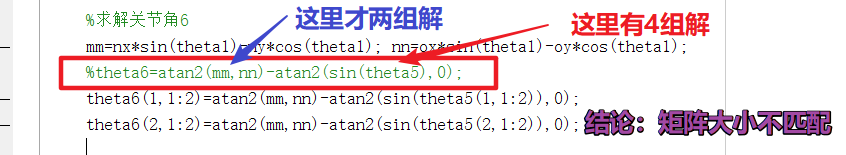同样的，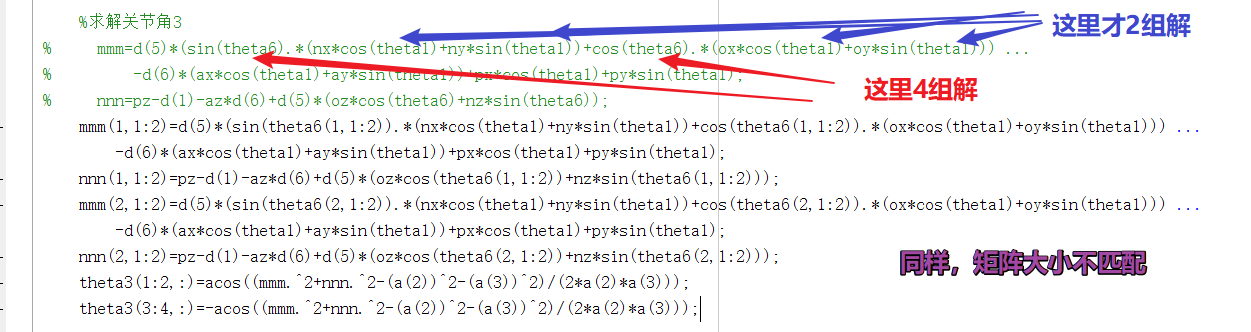改了这两个地方就好啦。这样，大家勉强能入门了，但如果能知其所以然，推导到其他标准DH系参数，就更好了！！！

展开全文Matlab UR5机械臂 6轴机械臂
• 2019/5/30 新编辑：已经有两个小伙伴证明了：我这个程序在我的MATLAB2017b上运行没问题，但在2018版本上就会报错，我也不知道是什么原因！！！ 2019/4/25新编辑：改正了zhengyundongxue和niyundon...


代码逻辑参考: https://blog.csdn.net/fengyu19930920/article/details/81144042#comments

2020/7/22补充：我把所有文件和机器人工具箱打包了，新来的朋友可以不往下看了，直接下载用吧。

链接：https://pan.baidu.com/s/

1n7uyCeUrw1zO1b7UGNDSnw

%%%%%%%%%%%%%%%%%%%%%自行把上面链接放到一行，进行搜索%%%%%%%%%%%%%%%%%%%%%
提取码：csdn

2020/7/22 补充：用机器人工具箱迭代求逆解有时报错，但niyundongxue.m求逆解没问题。所以有两种方法解决：1. 注释掉工具箱求逆解过程:

%  AA=ikine(robot_UR5,A);

2. 更换下theta角度值，比如更换为:

theta=[1,1/2,1/3,1,1,1];%没有什么含义，随便取的

此博客目的在于写个能求正逆解的MATLAB程序，便于其他功能开发，比较速成，被验证可行。

2019/5/30 新编辑：已经有两个小伙伴证明了：我这个程序在我的MATLAB2017b上运行没问题，但在2018版本上就会报错，我也不知道是什么原因！！！

2019/4/25 新编辑：改正了zhengyundongxue和niyundongxue中的DH表（之前写错了，汗。。。）

背景介绍：

本人想用    MATLAB+V-REP  仿真机器人，对机器人的正逆运动学简单写了下代码实现部分。

首先是正运动学求解代码（所用DH参数表为UR5）

function T = zhengyundongxue(theta)
%已知关节角求变换矩阵
a=[0,-0.42500,-0.39225,0,0,0];
d=[0.089159,0,0,0.10915,0.09465,0.08230];
alpha=[pi/2,0,0,pi/2,-pi/2,0];

T01=T_para(theta(1),d(1),a(1),alpha(1));
T12=T_para(theta(2),d(2),a(2),alpha(2));
T23=T_para(theta(3),d(3),a(3),alpha(3));
T34=T_para(theta(4),d(4),a(4),alpha(4));
T45=T_para(theta(5),d(5),a(5),alpha(5));
T56=T_para(theta(6),d(6),a(6),alpha(6));

T=T01*T12*T23*T34*T45*T56;

end

function T = T_para(theta,d,a,alpha)
T=[ccc(theta),-sss(theta)*ccc(alpha),sss(theta)*sss(alpha),a*ccc(theta);
sss(theta),ccc(theta)*ccc(alpha),-ccc(theta)*sss(alpha),a*sss(theta);
0,sss(alpha),ccc(alpha),d;
0,0,0,1];
end

好了，问题来了，这个ccc和sss函数就是cos和sin，我承认我SB了。。。其实我的目的是，在角度和弧度之间切换。

function sss=sss(a)
%     sss=sin(a/180*pi);
sss=sin(a);
end

function ccc=ccc(a)
%     ccc=cos(a/180*pi);
ccc=cos(a);
end

至此，正运动学代码部分贴完了。

其次，是逆运动学代码部分

function theta=niyundongxue(T)
%变换矩阵T已知
%SDH:标准DH参数表求逆解（解析解）
%部分DH参数表如下，需要求解theta信息

a=[0,-0.42500,-0.39225,0,0,0];
d=[0.089159,0,0,0.10915,0.09465,0.08230];

alpha=[pi/2,0,0,pi/2,-pi/2,0];% alpha没有用到,故此逆解程序只适合alpha=[pi/2,0,0,pi/2,-pi/2,0]的情况！

nx=T(1,1);ny=T(2,1);nz=T(3,1);
ox=T(1,2);oy=T(2,2);oz=T(3,2);
ax=T(1,3);ay=T(2,3);az=T(3,3);
px=T(1,4);py=T(2,4);pz=T(3,4);

%求解关节角1
m=d(6)*ay-py;  n=ax*d(6)-px;
theta1(1,1)=atan2(m,n)-atan2(d(4),sqrt(m^2+n^2-(d(4))^2));
theta1(1,2)=atan2(m,n)-atan2(d(4),-sqrt(m^2+n^2-(d(4))^2));

%求解关节角5
theta5(1,1:2)=acos(ax*sin(theta1)-ay*cos(theta1));
theta5(2,1:2)=-acos(ax*sin(theta1)-ay*cos(theta1));

%求解关节角6
mm=nx*sin(theta1)-ny*cos(theta1); nn=ox*sin(theta1)-oy*cos(theta1);
theta6=atan2(mm,nn)-atan2(sin(theta5),0);

%求解关节角3
mmm=d(5)*(sin(theta6).*(nx*cos(theta1)+ny*sin(theta1))+cos(theta6).*(ox*cos(theta1)+oy*sin(theta1))) ...
-d(6)*(ax*cos(theta1)+ay*sin(theta1))+px*cos(theta1)+py*sin(theta1);
nnn=pz-d(1)-az*d(6)+d(5)*(oz*cos(theta6)+nz*sin(theta6));
theta3(1:2,:)=acos((mmm.^2+nnn.^2-(a(2))^2-(a(3))^2)/(2*a(2)*a(3)));
theta3(3:4,:)=-acos((mmm.^2+nnn.^2-(a(2))^2-(a(3))^2)/(2*a(2)*a(3)));

%求解关节角2
mmm_s2(1:2,:)=mmm;
mmm_s2(3:4,:)=mmm;
nnn_s2(1:2,:)=nnn;
nnn_s2(3:4,:)=nnn;
s2=((a(3)*cos(theta3)+a(2)).*nnn_s2-a(3)*sin(theta3).*mmm_s2)./ ...
((a(2))^2+(a(3))^2+2*a(2)*a(3)*cos(theta3));
c2=(mmm_s2+a(3)*sin(theta3).*s2)./(a(3)*cos(theta3)+a(2));
theta2=atan2(s2,c2);

%整理关节角1 5 6 3 2
theta(1:4,1)=theta1(1,1);theta(5:8,1)=theta1(1,2);
theta(:,2)=[theta2(1,1),theta2(3,1),theta2(2,1),theta2(4,1),theta2(1,2),theta2(3,2),theta2(2,2),theta2(4,2)]';
theta(:,3)=[theta3(1,1),theta3(3,1),theta3(2,1),theta3(4,1),theta3(1,2),theta3(3,2),theta3(2,2),theta3(4,2)]';
theta(1:2,5)=theta5(1,1);theta(3:4,5)=theta5(2,1);
theta(5:6,5)=theta5(1,2);theta(7:8,5)=theta5(2,2);
theta(1:2,6)=theta6(1,1);theta(3:4,6)=theta6(2,1);
theta(5:6,6)=theta6(1,2);theta(7:8,6)=theta6(2,2);

%求解关节角4
theta(:,4)=atan2(-sin(theta(:,6)).*(nx*cos(theta(:,1))+ny*sin(theta(:,1)))-cos(theta(:,6)).* ...
(ox*cos(theta(:,1))+oy*sin(theta(:,1))),oz*cos(theta(:,6))+nz*sin(theta(:,6)))-theta(:,2)-theta(:,3);

end

代码测试部分：


L1 = Link('d', 0.089159, 'a', 0,        'alpha', pi/2 ,'standard' );
L2 = Link('d', 0,        'a', -0.42500,   'alpha',   0  ,'standard' );
L3 = Link('d', 0,        'a', -0.39225, 'alpha',   0  ,'standard' );
L4 = Link('d', 0.10915,  'a', 0,        'alpha', pi/2 ,'standard' );
L5 = Link('d', 0.09465,  'a', 0,        'alpha', -pi/2 ,'standard');
L6 = Link('d', 0.08230,  'a', 0,        'alpha',   0   ,'standard');

robot_UR5=SerialLink([L1,L2,L3,L4,L5,L6],'name','UR5');   %SerialLink 类函数
robot_UR5.display();  %Link 类函数
robot_UR5.teach(); %可以自由拖动的关节角度

theta=[1,1/2,1,1,1,1];%没有什么含义，随便取的

% robot_UR5.plot(theta);   %SerialLink 类函数

%利用机器人工具箱求正、逆运动学
A=fkine(robot_UR5,theta);
AA=ikine(robot_UR5,A);

%利用刚写的函数求正、逆运动学
B=zhengyundongxue(theta);
BB=niyundongxue(B);

结果部分：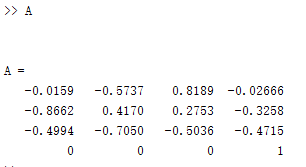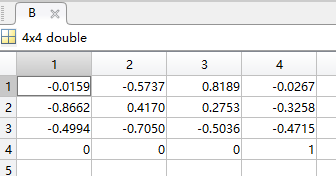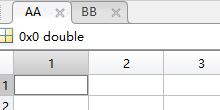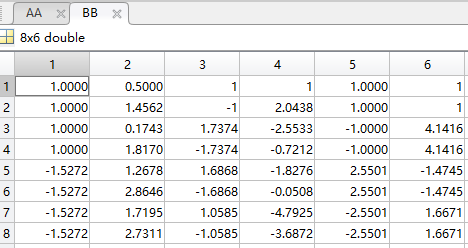经再次运行正运动学验证，我所求的8组逆解都是正确的。（不知道为什么机器人工具箱没有求出逆解？？？）

Tip：

1. 编写代码时，不要参考原博主https://blog.csdn.net/fengyu19930920/article/details/81144042#comments的结论部分，有错误，比如第一个m和n的定义与前述不同。

2. 关于奇异位置，未作说明。

3. 代码有没有硬核？名字无所谓，瞎起的，代码的逻辑不知道别人能不能看懂，能用就完事了。

4. 改进DH参数表求逆运动学，请参考https://blog.csdn.net/jldemanman/article/details/80785075，本人未验证她的代码是否可用（明天周五了，想了想这周进度，又是深深的难过。。。）

展开全文• 6. 正运动学标准DH参数matlab代码： clear; clc; syms theta1 theta2 theta3 theta4 theta5 theta6; syms alpha1 alpha2 alpha3 alpha4 alpha5 alpha6; syms a1 a2 a3 a4 a5 a6; syms d1 d2 d3 d4 d5 d6; T1 ...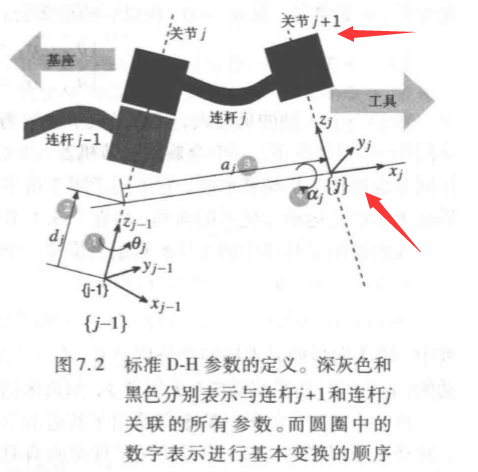1. 切记，{i}坐标系建立在i+1关节轴上，如{0}坐标系建立在关节1轴上，依次类推。{6}坐标系与{5}坐标系姿态一致，固连在法兰盘接口末端
2. 坐标系原点建立：若1 2轴垂直或异面垂直，则坐标系{1}原点在1轴与2轴的交点，{0}坐标系原点建在1轴与2轴的交点上，若1 2轴平行，则建立在公垂线与轴的交点上。
3. z轴与关节轴方向一致，Xi轴与Zi-1轴垂直且相交，其中X0轴方向指向1 2轴公法线方向，y轴由右手定则确定。
4. 参数确定： a为两Z轴距离，d为两x轴距离；alpha为两Z轴夹角，theta为两x轴夹角，方向判断如下图：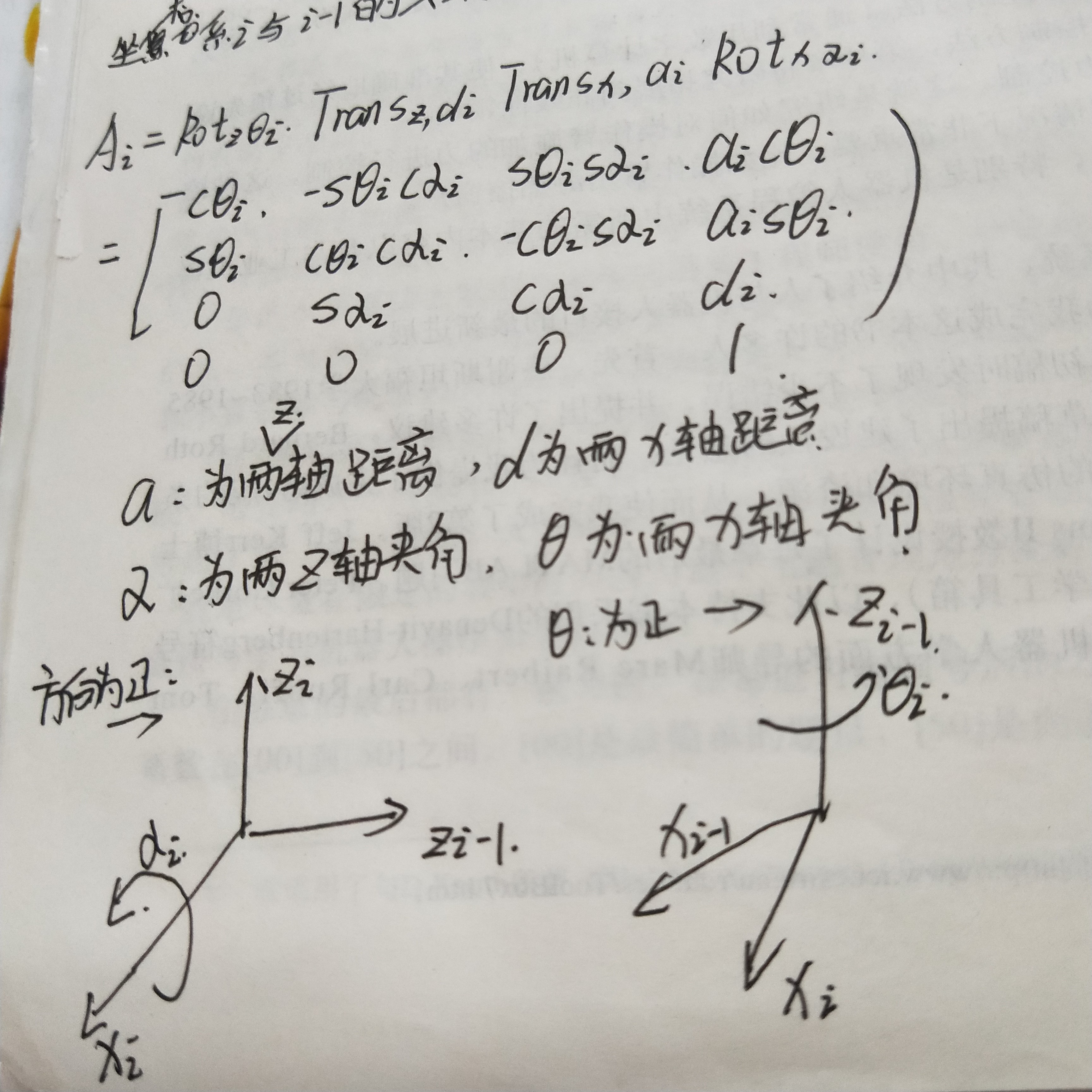5. 实例：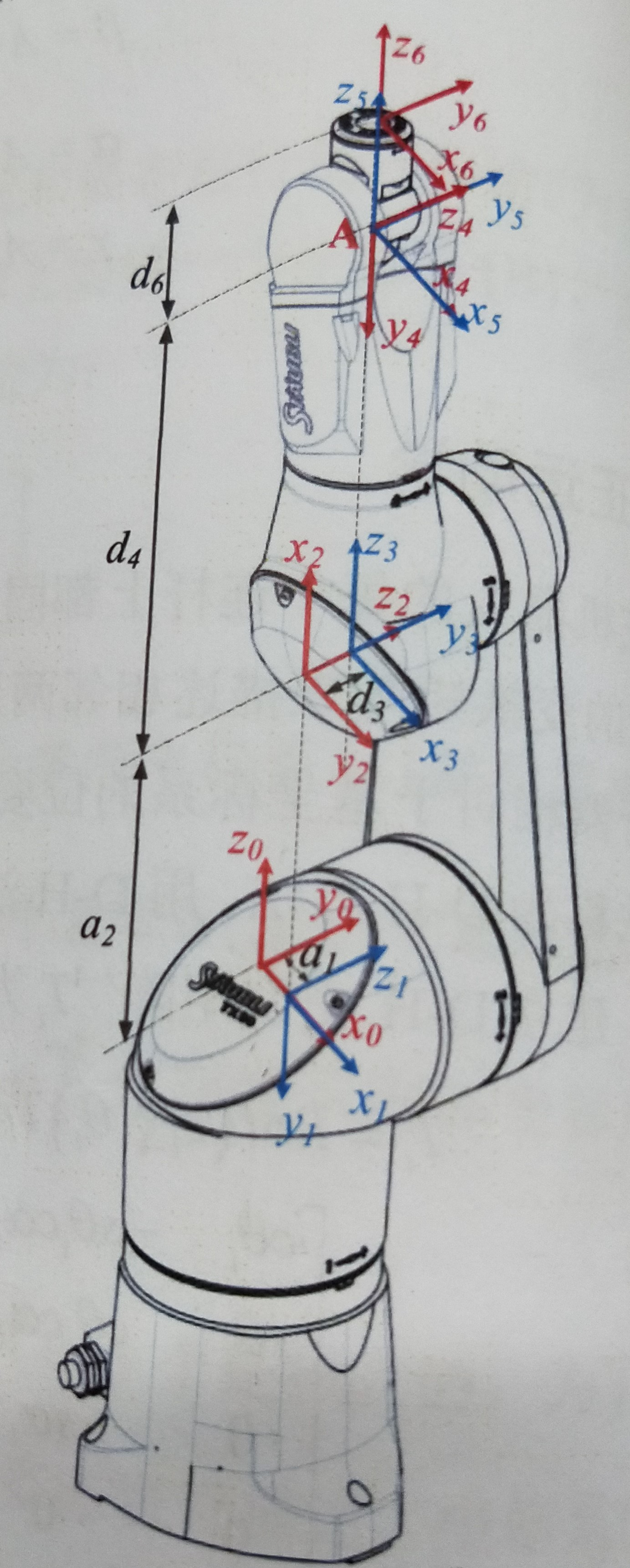6. 正运动学标准DH参数matlab代码：

clear;
clc;
syms theta1 theta2 theta3 theta4 theta5 theta6;
syms alpha1 alpha2 alpha3 alpha4 alpha5 alpha6;
syms a1 a2 a3 a4 a5 a6;
syms d1 d2 d3 d4 d5 d6;

T1 = [cos(theta1), -sin(theta1)*cos(alpha1), sin(theta1)*sin(alpha1), a1*cos(theta1);...
sin(theta1), cos(theta1)*cos(alpha1),  -cos(theta1)*sin(alpha1), a1*sin(theta1);...
0      ,       sin(alpha1),                cos(alpha1),      d1           ;...
0      ,             0         ,              0        ,       1           ];
T2 = [cos(theta2), -sin(theta2)*cos(alpha2), sin(theta2)*sin(alpha2), a2*cos(theta2);...
sin(theta2), cos(theta2)*cos(alpha2),  -cos(theta2)*sin(alpha2), a2*sin(theta2);...
0      ,       sin(alpha2),                cos(alpha2),      d2           ;...
0      ,             0         ,              0        ,       1           ];
T3 = [cos(theta3), -sin(theta3)*cos(alpha3), sin(theta3)*sin(alpha3), a3*cos(theta3);...
sin(theta3), cos(theta3)*cos(alpha3),  -cos(theta3)*sin(alpha3), a3*sin(theta3);...
0      ,       sin(alpha3),                cos(alpha3),      d3           ;...
0      ,             0         ,              0        ,       1           ];
T4 = [cos(theta4), -sin(theta4)*cos(alpha4), sin(theta4)*sin(alpha4), a4*cos(theta4);...
sin(theta4), cos(theta4)*cos(alpha4),  -cos(theta4)*sin(alpha4), a2*sin(theta4);...
0      ,       sin(alpha4),                cos(alpha4),      d4           ;...
0      ,             0         ,              0        ,       1           ];
T5 = [cos(theta5), -sin(theta5)*cos(alpha5), sin(theta5)*sin(alpha5), a5*cos(theta5);...
sin(theta5), cos(theta5)*cos(alpha5),  -cos(theta5)*sin(alpha5), a5*sin(theta5);...
0      ,       sin(alpha5),                cos(alpha5),      d5           ;...
0      ,             0         ,              0        ,       1           ];
T6 = [cos(theta6), -sin(theta6)*cos(alpha6), sin(theta6)*sin(alpha6), a6*cos(theta6);...
sin(theta6), cos(theta6)*cos(alpha6),  -cos(theta6)*sin(alpha6), a6*sin(theta6);...
0      ,       sin(alpha6),                cos(alpha6),      d6           ;...
0      ,             0         ,              0        ,       1           ];

T = simplify(eval(T1*T2*T3*T4*T5*T6));
nx = T(1,1)
ny = T(2,1)
nz = T(3,1)
ox = T(1,2)
oy = T(2,2)
oz = T(2,3)
ax = T(1,3)
ay = T(2,3)
az = T(3,3)
px = T(1,4)
py = T(2,4)
pz = T(3,4)
展开全文• ## DH参数的理解

千次阅读 2020-03-05 15:01:48
根据轴的先后顺序分为标准DH参数和改进DH参数。 标准DH参数：两个连杆坐标系变换是先绕z轴转动、平移，再绕x轴转动、平移。即X-Z顺序，先转动后平移。 改进DH参数：两个连杆坐标系变换是先绕X轴转动、平移，再绕Z轴...
• 这个使用标准 DH 参数，也可以在本书中找到： https : //www.cs.duke.edu/brd/Teaching/Bio/asmb/current/Papers/chap3-forward-kinematics.pdf 。 结果已通过Peter Corke的RVC工具箱（ ...matlab
• 机器人DH参数 DH的建模方法有两种，一种是标准的，一种是DH的； 对于标准DH建模，可以参考：《应用机器人学：运动学、动力学与控制技术》这本书。 对于改性的DH建模，可以参考：《机器人技术基础》 这两个的区别是：...
• 这篇文章介绍了建立机器人连杆坐标系的另外一种方法也就是修改DH参数法。这篇文章介绍了标准DH参数和修改DH参数的区别和联系。
• 文章目录一、前言1、描述两个坐标系的...二、标准DH参数建模三、修正DH参数建模四、实例(standard DH)1、SCARA机器人2、SCARA机器人 一、前言 1、描述两个坐标系的变换关系需要6个参数(3个表示位置变换，3个表示姿态...
• 这篇文章主要介绍了机器人连杆坐标系的建立方式即标准DH参数法建立坐标系。文章着重介绍了坐标系建立的过程以及各个参数的物理意义。连杆坐标系
• dh参数 1. 标准的STD_DH dh建模 Trans zi-1（di）:沿着zi-1轴平移di；Rot zi-1（）:沿着zi-1...标准DH参数和改进DH参数之间的差异是连接到连杆的坐标系的位置和齐次变换的顺序。 3.1标准的DH参数原点 Oi 坐...matlab
• 文章目录PUMA560四种参数标准DH和改进DHPUMA560的DH参数多的原因总结 PUMA560四种参数 链接:简直要崩溃了，PUMA560机器人DH参数表，你们见过多少个版本？. 先按这个同学列出的4个不同形式的参数来分析，我把这4个...
• Puma560机器人标准DH参数表如下所示 Theta:关节转角 D:连杆偏移量 A：连杆长度 Alpha：连杆扭转角 theta d a alpha 1 q1 0.0000 0.0000 90 2 q2 0.0000 0.4318(a2) 0 3 q3 0.1500(d3) 0.0203(a3) -90 4 q4人工智能
• 一般使用Robotics System Toolbox（RST）中的动力学仿真使用到的都是标准DH参数表，而我导入了改进型DH参数表。本来仿真的目的是求解四关节机器人的力矩（1关节是旋转关节，2,3关节是移动关节，4关节是旋转关节），...matlab
• 本文重点是建系以及提取DH参数表的过程 想要运行程序只需要把所有代码复制粘贴进matlab运行即可 1. 机器人结构 我这边选的机器人模型有点特殊，需要自行增加坐标系。这是因为这里的Tool frame不能用DH法 2.尝试用...机器人建模
• 仅供个人学习记录 DH法一般用一次就丢，然后后面再需要用的时候就...写出DH参数表 写出转换方程 其中对于第二步情况也要分为两种 旋转关节 平动关节 其中对于第四步情况分为三种： Zi与Zi-1不共面 Zi与Zi-1平行 ...机器人建模
• 标准DH与改进DH的区别如图： 标准（经典）DH是将连杆的坐标系固定在该连杆的输出端（下一关节），也即坐标系i-1与关节i对齐；改进DH是将连杆的坐标系固定在该连杆的输入端（同一关节），也即坐标系i-1与关节i-1...
• 产品型号:MC33394DH主要特性:提供5V,3.3V，2.6V线性输出总线类型和标准:高速CAN保护功能:限流，过热工作电压(V):4-26.5极限待机电流(uA)Typ/Max:20/50其它功能:多稳压器，看门狗，唤醒，休眠，停止方式诊断:SPI，4...
• 学习机器人建模并不是一个愉快的过程，不愉快的一个重要原因就是，建模得到的方程又臭又长，仅仅是计算一次也许都要花不少时间，更不要说除了正逆运动学方程，你还要需要动力学方程，甚至动力学参数标定方程。...机器人建模
• 这篇文章主要介绍了建立标准DH参数坐标系的一般流程以及其中的一些技巧。
• 使用标准 DH 参数描述的开放运动链的递归 Newton-Euler 逆动力学可选的： 机器人工具箱用于比较： http : //www.petercorke.com/RTB/ 下载并解压缩文件。 使用“pathtool”命令将路径添加到 MATLAB。 然后在 MATLAB ...matlab
• IRB2600的标准DH参数表： 轴号iii αi−1\alpha_{i-1}αi−1​ ai−1a_{i-1}ai−1​ did_{i}di​ θi\theta_{i}θi​ 1 0 0 d1(445)d_{1}(445)d1​(445) θ1\theta_{1}θ1​ 2 −90∘-90^{\circ}−90∘ a1...
• 本文档提供了详实的数据参数，特性，应用以及封装信息。带有数字I2C / SPI串行接口标准输出， 装置具有超低功耗运行模式允许先进的节电和智能嵌入式功能。LIS3DH具有动态用户可选的完整性scales2g /±4g /8g/16g...
•   （1）Link类函数，基于DH法建模，建立其相关关系，DH法建模分改进型和标准型，Link类函数的一种用法是 R = Link([theta,d,a, alpha])，其中参数theta代表DH建模的关节角、参数d代表DH建模的连杆偏距、参数a代表...matlab 机器人工具箱
• …改进的dh参数法… …总结一句话就是跟第一个杆件的重合… …同上也是和全一个重合…matlab
• ## 机械臂学习思路

千次阅读 2020-06-03 17:09:58
2020年初的时候，为了完成毕业设计的任务，开始尝试学习机器人...自由度的设置☞机械臂位姿的定义☞旋转与平移的概念☞齐次坐标与齐次变换的定义☞标准dh参数表和改进dh参数表的绘画与设计☞机械臂的运动学☞雅各比矩阵
• Eigen库可以实现matlab上类似的矩阵运算，非常方便，相关介绍参考后边链接，机器人正逆运动学求解...//师姐的参数，TX90，使用标准DH参数 void TX90robot::forward() { float theta1 = ntheta1*PI/180; float ...